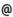## "Babes-Bolyai" University of Cluj-Napoca Faculty of Mathematics and Computer Science

 Mathematical analysis 1
 Code Semes-ter Hours: C+S+L Credits Type Section MMA0001 1 3+2+0 6 compulsory Matematică MMA0001 1 3+2+0 6 compulsory Matematică informatică MMA0001 1 3+2+0 6 compulsory Matematici aplicate
 Teaching Staff in Charge
 Prof. DUCA Dorel, Ph.D., dducamath.ubbcluj.ro
 Aims Getting to know the topology of the real axis and the differential and integral calculus of functions of one real variable.
 Content 1. Real Functions of one Real Variable The limit and the continuity of a real function of one real variable. The discontinuity points and their classification. Monotonuous functions. Functions with Darboux property. Uniformly continuous functions. Convex functions. 2. Differential Calculus The derivative and the differential of a real function of one real variable. Operations with derivable functions. The derivability of composed functions and of the inverse function. The fundamental theorems of differential calculus ( Fermat's theorem, Rolle's theorem, mean value theorems) and their consequences. The characterisation of the monotony with the help of the derivative. L'Hospital's rule. Higher order derivatives. The characterisation of the convexity with the help of the sign of the second order derivative. Taylor's formula. The characterisation of the optimum points with the help of the derivatives. Primitavible functions. The connection to the Darboux's property. 3. Integral Calculus in R Divisions of a compact, nondegenerat interval in R. The Riemann integrability of a function and the Riemann integral. The connections with the bounderies of a function. Properties of the Riemann integral. Darboux sums. The lower and higher integral of a bounded function. The connection of these with the integrability and the Riemann integral. The calculus of the Riemann integral. Newton-Leibniz's formula. The formula of integration through sides and the formula for the change of the variable. Criteria of Riemann integrability. Classes of Riemann integrable functions. Improper integrals. Criteria for their convergence. The calculus of improper integrals. 4. Sequences and series of functions Continuity, derivability and integrability properties of the limit function of a sequence of functions. Continuity, derivability and integrability properties of the sum of a series of functions. Power series. The set and the radius of convergence of a power series. Properties of the sum function. The expressing of a function through a power series.
 References 1. D. ANDRICA, D.I. DUCA, I. PURDEA, I. POP: Matematica de baza, Editura Studium, Cluj-Napoca, 2002 2. M. BALAZS, J. KOLUMBAN: Matematikai analizis, Dacia Konyvkiado, Kolozsvar-Napoca, 1978 3. W.W. BRECKNER: Analiză matematică. Topologia spaţiului Rn, Universitatea din Cluj-Napoca, Cluj-Napoca, 1985 4. S. COBZAS: Analiza matematica (Calcul diferential), Presa Universitara Clujeana, Cluj-Napoca, 1997 5. D.I. DUCA, E. DUCA: Analiza matematica. Culegere de probleme, Editura GIL, Zalau, 1999 6. L. LUPSA, L. BLAGA: Analiza matematica. Note de curs 1, Presa Universitara Clujeana, Editura Mega, Cluj-Napoca, 2003 7. H. LUENBURG: Vorlesungen uber Analysis, Manheim, Bibliographisches Institut, 1981 8. M. MEGAN: Bazele Analizei matematice, vol. 1,2,3, Editura Eurobit, 1997, 1997, 1998 9. GH. SIRETCHI: Calcul diferential si integral, vol. I si II, Editura Stiintifica si Enciclopedica, Bucuresti,1985 10. T. TRIF: Probleme de calcul diferential si integral in Rn, Casa Cartii de stiinta, Cluj-Napoca, 2003
 Assessment The activities from the seminary and a written paper during the semester (conting for 20% of the final grade) and a written exam at the end of the semester (counting for 80% of the final grade).